## 31 Mar 2016

### Agler (2.1) Symbolic Logic: Syntax, Semantics, and Proof, "Truth Functions," summary

by Corry Shores
[Search Blog Here. Index-tags are found on the bottom of the left column.]

[The following is summary. Boldface, underlining, and bracketed commentary are my own. I highly recommend Agler’s excellent book. It is one of the best introductions to logic I have come across.]

Summary of

David W. Agler

Symbolic Logic: Syntax, Semantics, and Proof

Ch.2: Language, Syntax, and Semantics

2.1 Truth Functions

Brief Summary:
We will examine the language of propositional logic (PL). Propositions with no truth functional operators are are atomic propositions. A propositional operator makes a proposition more complex. If it does so by combining propositions, then it is a propositional connective. Propositional operators are truth-functional if the value of the more complicated proposition they make is entirely dependent on the truth value of the component parts.

Summary

Ch.2: Language, Syntax, and Semantics

In this chapter, we will examine the formal language of propositional logic (PL).

2.1 Truth Functions

In English, propositions can be built up from propositional connectives like and, or, if ... then, and if and only if (25). Agler provides this definition:

Propositional connective: A propositional connective is a term (e.g., and, or, if ... then..., ... if and only if ...) that connects or joins propositions to create more complex propositions.
(26)

Agler then notes how we can modify sentences in ways that make more complex propositions but without connecting one proposition with another. He offers these examples:

(1) John went to the store.
(7) It is not the case that John went to the store.
(8) It is known that John went to the store.
(9) It is suspected that John went to the store.
(Agler 26)

This other kind of modification are called propositional operators:

Propositional operator: A propositional operator is a term (e.g., and, or, it is not the case that) that operates on propositions to create more complex propositions.
(26)

In this book, we are concerned mostly with propositional operators that are used truth-functionally. He explains:

A propositional operator is used truth-functionally insofar as the truth value of the complex proposition is entirely determined by the truth values of the propositions that compose it. Propositional operators that are used truth-functionally are called truth-functional operators.

Truth-functional operator: A truth-functional operator is a propositional operator (e.g., and, or, it is not the case that) that is used in a truth-functional way.
(26)

Agler now distinguishes two kinds of propositions. The first kind are atomic propositions.

Atomic proposition: An atomic proposition is a proposition without any truth-functional operators.
(26)

Atomic propositions do not have any logical operators. The second kind are complex propositions.

Complex proposition: A proposition is complex when it has at least one truth-functional operator.
(27)

We may understand how truth-functional operators work by noting how logical functions work: “In general, a function associates a value or values (known as the input) with another value (known as the output)” (27). The specific sort of value that concerns us is are truth values, and thus we are interested in truth-value functions.

Truth function: A truth function is a kind of function where the truth-value output is entirely determined by the truth-value input.
(28)

One example where “The truth value of the output proposition is entirely determined by the truth values of the input propositions” is conjunction (28).

Agler, David. Symbolic Logic: Syntax, Semantics, and Proof. New York: Rowman & Littlefield, 2013.

### Lawvere & Schanuel (Preview) Conceptual Mathematics: A First Introduction to Categories, “Galileo and Multiplication of Objects”, summary

by Corry Shores
[Search Blog Here. Index-tags are found on the bottom of the left column.]

[The following is summary. All boldface and bracketed commentary is mine.]

Entry Directory for

F. William Lawvere & Stephen H. Schanuel

Conceptual Mathematics: A First Introduction to Categories

Preview

Session 1: Galileo and Multiplication of Objects

Brief Summary:

In this book we explore the notion of category in terms of objects, maps, and map composition. We are here first given a glimpse with the example of maps that “multiply” domains, as in the case of motion being decomposed into domains of temporal and spatial locations, the latter of which being decomposed into multiple spatial dimensions.

Summary

1. Introduction

In this book, the authors will “explore the consequences of a new and fundamental insight about the nature of mathematics which has led to better methods for understanding and using mathematical concepts” (3). They continue:

The basic notion which underlies all the others is that of a category, a ‘mathematical universe’. There are many categories, each appropriate to a particular subject matter, and there are ways to pass from one category to another.
(3)

So they will begin with an informal introduction that will provide examples of objects, maps, and composition of maps.

Although these notions were used informally one way or another throughout the history of mathematics, it was not until 1945 that Eilenberg and MacLane gave explicit definitions to these basic concepts (3)

2. Galileo and the Flight of a Bird

Galileo wanted to understand the motion of objects. Motion is more than just the track of locations an object makes, like the parabolic arc of a thrown rock. “The motion involves, for each instant, the position of the rock at that instant; to record it requires a motion picture rather than a time exposure. We say the motion is a ‘map’ (or ‘function’) from time to space” (3).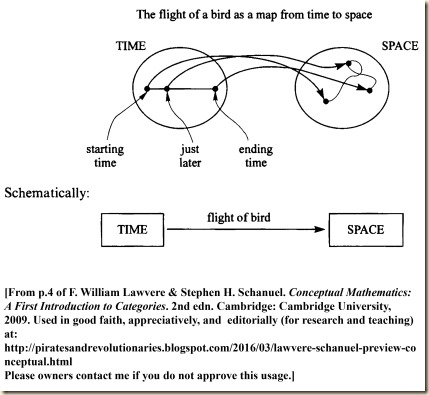As the legend goes, Galileo dropped both a heavy and a light weight simultaneously from the leaning tower of Pisa. Spectators were surprised to see that they both landed at the same time. But this motion is not so interesting, because the object moves in a straight line. The reason the authors say Galileo chose it has to do with the simple equation:

SPACE = PLANE × LINE

The authors will now explain.

We will consider two new maps. We first are to imagine that the sun is directly overhead. So for each point in space [intervening between us and the sun] we will have a shadow point on the horizontal plane [the ground below us].This shadow map from space to the plane is our first map. Now for the second map, we need to think of a pole sticking upright in the ground, because we need now a vertical line. [So the horizontal plane gives us the coordinates of the point as they spread out horizontally, but without indication of how high or how low the point is. The vertical line will now indicate how high or low, but not where the point is on a horizontal plane.]

The second map we need is best imagined by thinking of a vertical line, perhaps a pole stuck into the ground. For each point in space there is a corresponding point on the line, the one at the same level as our point in space. Let’s call this map ‘level’:
(3)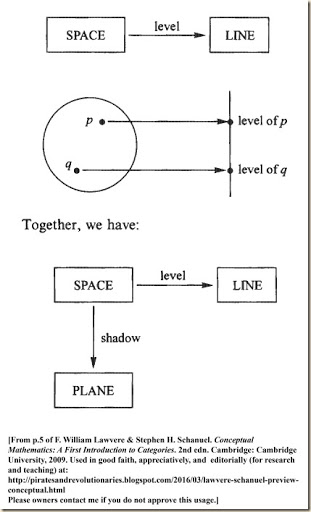So now we have two maps, the “shadow” map and the “level” map.  Together these two maps “seem to reduce each problem about space to two simpler problems, one for the plane and one for the line” (5). So we can determine a bird’s location in our space by knowing where its shadow is and what level it is at. [The next example is more complicated. For this we will trace the entire path of a bird flying through the space. We need two cameras for this, but how it all works out I am not sure. We need one camera-person who keeps aimed at the flying bird, but this camera-person moves up and down, staying level with the bird. Simultaneously we need another camera-person filming the first camera-person. I do not know how the footage will be combined to trace the flight, however. How does the level-changing camera-person capture the horizontal position? Would she not need to be above the bird to know the horizontal position, since the plane is two dimensional? Let me quote:]

Suppose you have a motion picture of the bird’s shadow as it flies, and a motion picture of its level – perhaps there was a bird-watcher climbing on our line, keeping always level with the bird, and you filmed the watcher. From these two motion pictures you can reconstruct the entire flight of the bird! So not only is a position in space reduced to a position in the plane and one on the line, but also a motion in space is reduced to a motion in the plane and one on the line.
(5)

[Recall this chart from before:This very generally gave us a relation between the time and space of the bird’s flight. We then decomposed the spatial component into two parts, the horizontal plane (which has two dimensions) and the vertical part (with one dimension). The authors will show how the original double mapping of time and space, when combined with the double mapping of space to plane and line, makes a triple mapping that then compresses to a double map of time to plane and line. ]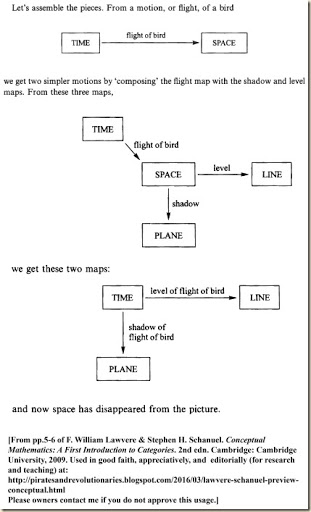Using this mapping, Galileo was able “to reduce the study of motion to the special cases of horizontal and vertical motion” (6).

The authors then ask if it is reasonable to use the equation SPACE = PLANE × LINE to express the relation of the two maps in this diagram.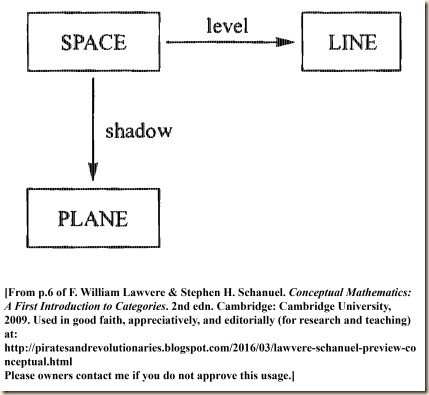They then wonder, “What do these maps have to do with multiplication?” To answer this, they will examine more examples.

3. Other Examples of Multiplication of Objects

They say that multiplication “often appears in the guise of independent choices” (7). They offer the example of option lists on restaurant menus. There may be a number of courses, and we choose an option from one list for the first course, and then another option from another list for the second course. A meal is comprised of one item from each list. The options for the first course are: soup, pasta, or salad. And for the second course: steak, veal, chicken, or fish.

We could for example make a meal as “soup, then chicken.” But one meal we cannot make is “veal, then steak” [since they are both on the same list, and nothing was chosen from the other list] (7). The authors then provide this diagram for possible meals, and they also show the mapping is comparable to the Galileo chart: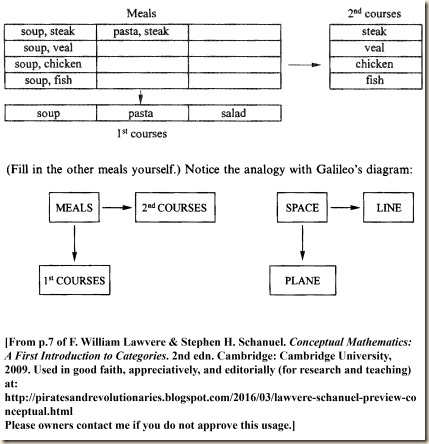So here we have a scheme with three “objects” and two “maps” or “processes”. This is a multiplication of objects. We find it operating in many different situations. In fact, a cylinder can be understood as the multiplication of a disc with its various levels. This is not just finding volume by multiplying these dimensions. Rather, the cylinder is composed by a process of multiplying the components.

Take for example a segment and a disk from geometry. We can multiply these too, and the result is a cylinder. I am not referring to the fact that the volume of the cylinder is obtained by multiplying the area of the disk by the length of the segment. The cylinder itself is the product, segment times disk, because again there are two processes or projections that take us from the cylinder to the segment and to the disk, in complete analogy with the previous examples. | Every point in the cylinder has a corresponding ‘level’ point on the segment and a corresponding ‘shadow’ point in the disk, and if you know the shadow and level points, you can find the point in the cylinder to which they correspond. As before, the motion of a fly trapped in the cylinder is determined by the motion of its level point in the segment and the motion of its shadow point in the disk.
(7-8)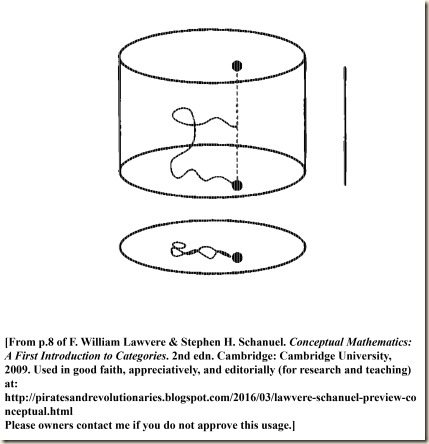The authors then suggest that we can understand multiplication in terms of the conjunctive operator in logic, “and” (8). We will take a conjunction of the form, A and B, for example, “John is sick and Mary is sick” (8). From this we can deduce A and as well we can deduce B.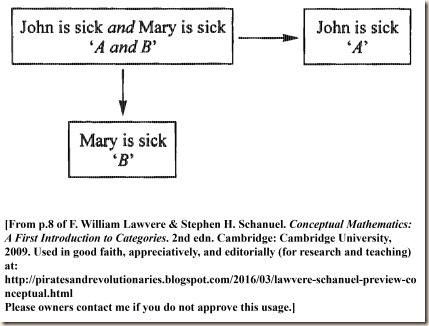[The next point I do not follow so well. It seems they are are saying that we already inferred the original “John is sick and Mary is sick” from yet another sentence, but I am not sure. Let me quote so you can interpret it:]

But more than that: to deduce the single sentence ‘John is sick and Mary is sick’ from some other sentence C is the same as deducing each of the two sentences from C. In other words, the two deductions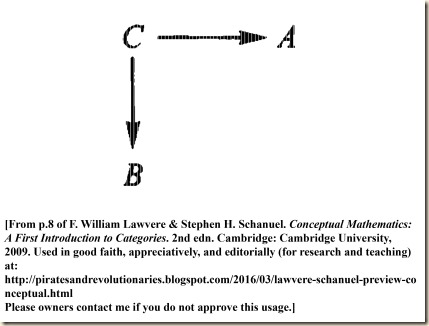amount to one deduction C → (A and B). Compare this diagram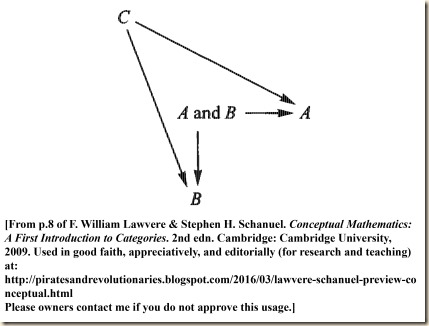with the Diagram of Galileo’s idea.
(8)

The authors then give one last diagram, this time for numerical multiplication.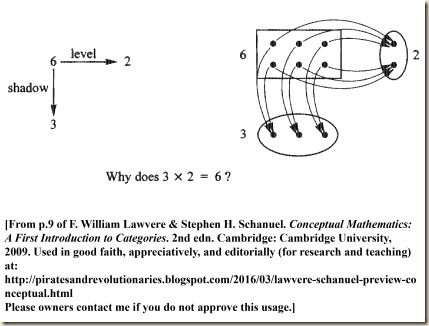The authors conclude by writing: “I hope these pictures seem suggestive to you. Our goal is to learn to use them as precise instruments of understanding and reasoning, not merely as intuitive guides” (9).

F. William Lawvere & Stephen H. Schanuel. Conceptual Mathematics: A First Introduction to Categories. 2nd edn. Cambridge: Cambridge University, 2009 [first published 1997].

.

### Lawvere & Schanuel’s Conceptual Mathematics: A First Introduction to Categories, entry directory

by Corry Shores
[Search Blog Here. Index-tags are found on the bottom of the left column.]

Entry Directory for

F. William Lawvere & Stephen H. Schanuel

Conceptual Mathematics: A First Introduction to Categories

Preview

Part I: The Category of Sets

Article 1: Sets, Maps, Composition: A First Example of a Category

F. William Lawvere & Stephen H. Schanuel. Conceptual Mathematics: A First Introduction to Categories. 2nd edn. Cambridge: Cambridge University, 2009 [first published 1997].

.

### Wikibooks: Set Theory, “Ordered Pairs”, summary

[Search Blog Here. Index-tags are found on the bottom of the left column.]

[Central Entry Directory]
[Logic & Semantics, Entry Directory]
[Set Theory, entry directory]

[Wikibooks: Set Theory, entry directory]

[My commentary is in brackets.]

Summary of

Wikibooks: Set Theory

Relations

Ordered Pairs

Brief summary:
In a set, the order of the members does not matter. But since we will want to work with relations, we will need groupings where the order does matter. We notate an ordered pair as (a, b) [rather than with curly braces, as with sets], and here the order of the terms does matter.

Summary

[Previously we were dealing with sets where the order of the members did not matter. Recall that the set was defined in the following way: “A mathematical set is defined as an unordered collection of distinct elements.” But now we will discuss relations of  sets. This will mean that one set will be related to another or to others. With that in mind, when notating this relation, the order will matter, since not all relations are symetrical. I am not sure, but in the ordered pairs we work with, we will drop the set braces, so they perhaps are not sets strictly speaking. So to understand the first formulation they will give, consider sets a, b, c, and d. And suppose that a=d and b=c. We put them into these pairings: (a, b) and (c, d). Now, if ordered mattered in the pairings, then these two pairings would not be equal, because the a is in the first position but the d is in the second. Now suppose instead that a=c and b=d. And we keep the same pairings: (a, b) and (c, d). Now we can say they are equal: (a, b) = (c, d).]

To define relations on sets we must have a concept of an ordered pair, as opposed to the unordered pairs the axiom of pair gives. To have a rigorous definition of ordered pair, we aim to satisfy one important property, namely, for sets a,b,c and d,.
(Wikibooks)

[The next part I do not understand very well; but let me quote it first, and then I will discuss it.]

As it stands, there are many ways to define an ordered pair to satisfy this property. A simple definition, then is. (This is true simply by definition. It is a convention that we can usefully build upon, and has no deeper significance.)

TheoremProof

Ifand, then.
Now, ifthen. Then, soand a=c.
So we have. Thusmeaning.

If, we haveand thusso.
If, note, so(Wikibooks)

[I did not understand the arbitrary definition of (a, b) = {{a},{a,b}}. After searching a bit, I found an excellent explanation by Arturo Magidin. The main idea is that it is not a definition in the sense that we normally think of them. The formulation {{a},{a,b}} does not intuitively present conceptual content that helps us understand why the order of the terms holds in (a, b). Rather, this definition of (a, b) as {{a},{a,b}} happens to allow us to form a proof for

(a, b) = (c, d) ↔ a=cb=d

. So all that is important is how this definition works in the proof, and not how it helps us understand the property itself. Here is the explanation by Arturo Magidin:

This definition is part of a way to try to define a lot of the things that we use in mathematics on the basis of an axiomatic theory; in this case, we start with Axiomatic Set Theory, where the only notions we have (if we are working in Zermelo-Fraenkel Set Theory) are “set” and “is and element of”, together with the axioms that tells us properties of sets and things we can do with sets.

We want to have something that works like what we know as "the ordered pair"; but all we have to work with are sets. So we need to find a way of constructing a set that has the properties we want for the ordered pair.

For a metaphor: the ordered pair is like a car; we know how to drive. But in order to actually have a car, there needs to be an engine and gasoline, and the engine has to work. We are trying to construct that engine so that we can later drive it.

So this is not notation, this is a definition of what the ordered pair is in set theory. We are defining an object, which we call “(a,b)”, to be the given set. It's not merely how we are writing the ordered pair, is what the ordered pair is if you are interested in actually seeing the engine of the car working. We know what we want “ordered pair” to behave like, but we have to actually construct an object that behaves that way. This is a way of defining an object that does behave that way.

There aren’t “two notations” here. We define “the ordered pair with first component a and second component b” to be the set

{{a},{a,b}},

(which one can prove is indeed a set using the Axioms of Set Theory, if a and b are already in the theory).

Then we prove that “the ordered pair with first component a and second component b” is equal to “the ordered pair with first component c and second component d” if and only if a=c and b=d.

Then we abbreviate “the ordered pair with first component a and second component b” by writing “(a,b)” (or sometimes “⟨a,b⟩”).

“(a,b)” is notation. The other side is the definition of this set.

The definition is the way it is because it works; that’s really all we care about. In fact, we forget about the definition pretty much as soon as we can, and simply use the (a,b) and the “defining property.” We can do that, because we know that “under the hood” there actually is an engine that does what we need it to do, even if we don’t see it working while we are driving the car.

So, there is only one bit of notation, and it's “(a,b)”. The other side is the definition of what that notation actually is.
(Arturo Magidin at math.stackexchange.com)

]

Source (of text and images):

Wikibooks. “Set Theory/Axioms.” <https://en.wikibooks.org/wiki/Set_Theory/Relations>
(Accessed 27-03-2016)

Or if otherwise noted:

http://math.stackexchange.com/questions/62908/how-can-an-ordered-pair-be-expressed-as-a-set

.

### Agler (1.3-1.4) Symbolic Logic: Syntax, Semantics, and Proof, "Deductively Valid Arguments," summary

by Corry Shores
[Search Blog Here. Index-tags are found on the bottom of the left column.]

[The following is summary. Bracketed commentary is my own. I highly recommend Agler’s book.]

Summary of

David W. Agler

Symbolic Logic: Syntax, Semantics, and Proof

Ch.1: Propositions, Arguments, and Logical Properties

Section 1.3: Deductively Valid Arguments
and
Section 1.4: Summary

Brief Summary:
An argument is deductively valid if it is impossible for the premises to be true and the conclusion false, and it is invalid otherwise. An argument is sound if the premises in fact are true and as well it is valid. It is unsound if either it is invalid or if any of the premises are false.

Summary

1.3 Deductively Valid Arguments

Elementary symbolic logic is concerned primarily with deductively valid arguments (14).

1.3.1 Deductive Validity Defined

Deductive validity applies only to arguments (15).

There are two ways to define deductively validity, both given below:

Validity: An argument is deductively valid if and only if, it is necessarily the case that if the premises are true, then the conclusion is true. That is, an argument is deductively valid if and only if it is logically impossible for its premises/assumptions to be true and its conclusion to be false.
(16)

We thus define deductive invalidity in the following way:

Invalidity: An argument is deductively invalid if and only if the argument is not valid.
(16)

We now recall the second formulation: “an argument is deductively valid if and only if it is logically impossible for its premises/assumptions to be true and its conclusion to be false” (16). We now need to define this impossibility. [Something is logically impossible if it involves a logical contradiction, and there is a contradiction among propositions if they are false no matter what the circumstances may be. Thus, if the premises in combination with the conclusion are false under any possible circumstance, then the inference leading to that conclusion is invalid.]

Impossibility: Something is logically impossible if and only if the state of affairs it proposes involves a logical contradiction.

A proposition is a logical contradiction if and only if, no matter how the world is, no matter what the facts, the proposition is always false.

Contradiction: A proposition is a contradiction that is always false under every circumstance.
(16)

Agler illustrates with these examples:

(1) John is 5'11, and John is not 5'11.

(3) Frank is the murderer, and Frank is not the murderer.
(Agler 16)

As we can see, sentences 1-3 are false, because no matter how we imagine the world, they cannot be true.  “Since the state of affairs they propose involves a contradiction, each one of these is logically impossible. That is, | under no situation, circumstance, or way the world could be can John be two different heights, can Toronto be in Canada and not in Canada, or can Frank be the murderer and not be the murderer” (16).

And as we noted, if it is impossible [that is, if it leads to a contradiction] that the premisses are true and the conclusion is false, then the inference is valid: “we would be uttering something contradictory (always false) if we were to say that a deductive argument’s premises/assumptions were true and its conclusion was false” (17).

1.3.2 Testing for Deductive Validity

Given the above definition and explanation of deductive validity and deductive invalidity, in order to test an inference as to which it is, we can ask the following question:

Is it logically impossible for the premises to be true (condition 1) and the conclusion to be false (condition 2)?
(17)

Agler then provides a step-by-step procedure for testing validity in this way, called the Negative Test for Validity. The steps are depicted in the chart below: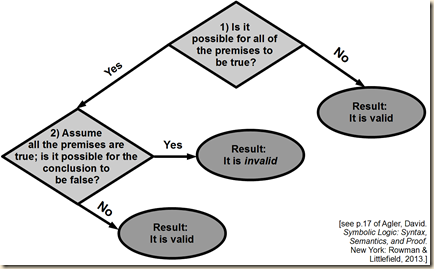So let us consider some possibilities to illustrate.

(1) All men are mortal.
(2) Barack Obama is a man.
(3) Therefore, Barack Obama is mortal.
(Agler, 17)

Is it possible for all the premises to be true? Certainly (for in fact they are true). Assuming them to be true, is it also possible for the conclusion to be false? No it is not, because this leads to a contradiction. [If Barack Obama is a man, and all men are mortal, it cannot be that Barack Obama is not mortal.]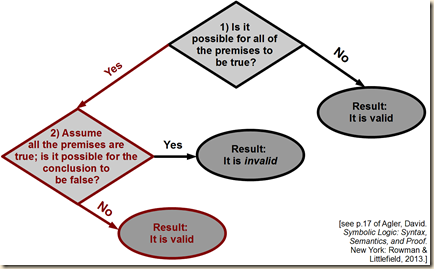Consider now these propositions:

(1) Some horses are domesticated.
(2) All Clydesdales are horses.
(3) Therefore, all Clydesdales are domesticated.
(Agler, 19)

Is it possible for all the premises to be true? Yes, it is. Assuming them to be true, is it also possible for the conclusion to be false? In fact, yes, it is. [If it said, “All horses are domesticated”, then the situation would be different. In that case, it would be impossible for both the premises to be true the conclusion to be false. But as it is written in the example above, it is possible for the conclusion to be false. For, Clydesdales could be a subgroup of horses that are not domesticated, since only some horses are.]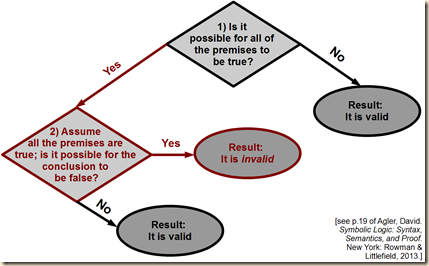[Agler then given an example for when all the premises are false, but the conclusion is true:

(1) All humans are donkeys.
(2) James the donkey is human.
(3) James the donkey is a donkey.
(Agler, 19)

But I am not sure that this is an instance that illustrates the other case we have not addressed, where it is impossible for the premises to be true. I can imagine that new evolutionary evidence is discovered that tells us that humans are a species of donkey. In other words, I do not see a logical contradiction among the premises. It might be helpful to consider an inference  that Graham Priest offers in Ch.2 of his book Logic: A Very Short Introduction:

(1) The Queen is rich.
(2) The Queen is not rich.
(3) Therefore, pigs can fly.
(Priest, 7)

Here it is not possible for the premises to be true, because that leads to a contradiction. Therefore, the inference is valid, even though the conclusion is false.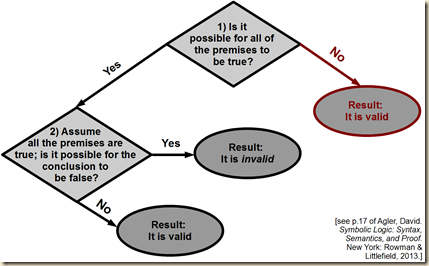]

On of the valid inferences [which I skipped] was:

(1) All men are immortal.
(2) Barack Obama is a man.
(3) Therefore, Barack Obama is immortal.
(Agler 18)

What is notable here is that premise (1) is false. No man is immortal. Even so, the whole inference is valid. In this case, we would say that it is valid but unsound. Agler explains:

Deductive arguments can either be valid or invalid, and if they are valid, they can be sound or unsound. An argument is sound if and only if it is both valid and all of its premises are true.

Sound: An argument is sound if and only if it is valid and all of its premises are true.

One way of thinking about soundness is through the following formula:

Validity + all true premises = sound argument

An argument is not sound (or unsound) in either of two cases: (1) if an argument is invalid, then it is not sound; (2) if an argument is valid but has at least one false premise, then it is not sound.

Unsound: An argument is unsound if and only if it is either invalid or at least one premise is false.
(20)

Logic can determine whether or not an argument is valid. But whether each premise is true or false is something formal logic is mostly unable to  do. So, “if a premise is contingent (one whose truth or falsity depends upon the facts of the world), then its logical form does not tell us whether the premise is true or false, and while the argument may be valid, we will not be able to determine whether or not it is sound without empirical investigation” (20).

1.4 Summary

Agler summarizes how we met our stated goals in this chapter, namely, we discussed propositions, arguments, and deductive validity (20).

Agler, David. Symbolic Logic: Syntax, Semantics, and Proof. New York: Rowman & Littlefield, 2013.

Or if otherwise noted:

Priest, Graham. Logic: A Very Short Introduction. Oxford: Oxford University, 2000.

### Agler (Intro, 1.1-1.2) Symbolic Logic: Syntax, Semantics, and Proof, summary

by Corry Shores
[Search Blog Here. Index-tags are found on the bottom of the left column.]

[The following is summary. Bracketed commentary is my own. I highly recommend Agler’s book.]

David W. Agler

Symbolic Logic: Syntax, Semantics, and Proof

Introduction

and

Ch.1: Propositions, Arguments, and Logical Properties

Sections 1.1-1.2: Propositions – Arguments

Summary

Brief Summary:
Logic studies the structures and mechanics of correct reasoning that takes an argumentative form. An argument is made of premises that lead to some conclusion. An argument may be (a) inductive, meaning that the conclusion is based on probabilities discerned from the premises, (b) abductive, meaning that the conclusion provides the best explanation (or theory) to account for the premises, or (c) deductively valid, meaning that the conclusion follows by logical necessity from the premises.

I.1 What is Symbolic Logic?

Symbolic logic is a branch of logic that represents how we ought to reason by using a formal language consisting of abstract symbols. These abstract symbols, their method of combination, and their meanings (interpretations) provide a precise, widely applicable, and highly efficient language in which to reason.
(1)

I.2 Why Study Logic?

Logic has a variety of applications, including learning circuit design, learning other formal languages (as with computer programming), developing skills in rule-following behavior, developing problem- solving and analytic skills, and enhancing a study of philosophy.

I.3 How Do I Study Logic?

You learn logic through study and practice.

I.4 How is the Book Structured?

Part I provides basic concepts like argument and validity. Part II describes propositional logic. Part III examines predicate logic, including formal proofs and semantics.

Chapter 1:
Propositions, Argument, and Logical Properties

“Logic is a science that aims to identify principles for good and bad reasoning” (5). It is prescriptive: it tells us how we should reason. The two main concepts of logic are propositions and arguments (5).
Propositions are the bearers of truth and falsity, while an argument is a series of propositions separated by those that are premises (or assumptions) and those that are conclusions.
(5)

1.1 Propositions

Agler defines proposition this way:
Proposition: A proposition is a sentence (or something expressed by a sentence) that is capable of being true or false.
(6)

Certain sentences may or may not be propositions, because it can depend on their use in context (6). But for the most part, commands, questions, and exclamations do not constitute propositions (7).

1.2 Arguments

1.2.1 What is an Argument?

Here is Agler’s definition of an argument:
Argument: An argument is a series of propositions in which a certain proposition (a conclusion) is represented as following from a set of premises or assumptions.
(7)

Some arguments start with assumptions, which are “propositions that are not claimed to be true but instead are supposed to be true for the purpose of argument” as with reductio ad absurdum arguments (8).

1.2.2 Identifying Arguments

Agler explains that if we are looking for arguments in everyday discourse, we can look for these two important features:
1) Arguments often have “argument indicators” that tell us there are inferential steps, like “therefore,” “in conclusion”, and
2) Often there is a conclusion that one is claiming follows from the premises or assumptions.
(Agler 8)

1.2.3 Argument Indicators

Agler lists the following argument indicators: Therefore, I infer that, Since, So, It follows that, Hence, In conclusion, For the reason that, Thus, Consequently, Inasmuch as, We deduce that, It implies, Ergo, We can conclude that (9).

1.2.4 Types of Arguments

There are different ways that the conclusion will follow from the premisses. In other words, there are different general types of inference.
1) Inductive argument: “The premises provide some degree of support for the conclusion but certainly do not guarantee the truth of the conclusion” (10). For example, on the table is a brown bag full of beans. We pull out one bean at a time. The first hundred times we pull out a black bean. We then conclude that the next bean will be black.
2) Abductive argument: Here “the truth of the premises does not guarantee the truth of the conclusion or even provide direct support for the conclusion.” Nonetheless, “the conclusion would (perhaps best) explain the premises” (11a). For example, imagine now that there is a closed brown bag on the table. Next to it are a bunch of black beans. We then conclude from these two facts that the beans came from the brown bag. [This sort of reasoning is like proposing the best theory to explain a set of facts.]
3) Deductively valid argument: Here “the truth of the premises guarantees the truth of the conclusion. That is, it is necessarily the case that, if the premises are true, then the conclusion is true. In other words, it is logically impossible for the premises to be true and the conclusion false” (11). Agler  gives this example, “If John is a crooked lawyer, then he will hide evidence. John is a crooked lawyer. Therefore, John will hide evidence” (11).

We should note also that a conclusion follows from its premises in a way that is different from how events follow from one another chronologically (11-12).

Agler, David. Symbolic Logic: Syntax, Semantics, and Proof. New York: Rowman & Littlefield, 2013.

.

### Agler’s Symbolic Logic: Syntax, Semantics, and Proof, entry directory

by Corry Shores
[Search Blog Here. Index-tags are found on the bottom of the left column.]

A version with the inclusion of brief summaries and examples can be found here:

Agler’s Symbolic Logic: Syntax, Semantics, and Proof, collected brief summaries [with examples]

Entry Directory for

David W. Agler

Symbolic Logic: Syntax, Semantics, and Proof

Introduction / Ch.1:

Ch.2: Language, Syntax, and Semantics

2.2 The Symbols of PL and Truth-Functional Operators

2.3 Syntax of PL

2.4 Disjunction, Conditional, Biconditional

Ch.3: Truth Tables

3.1 Valuations (Truth-Value Assignments)

3.2 Truth Tables for Propositions

3.3 Truth Tables Analysis of Propositions

3.4 Truth Tables Analysis of Sets of Propositions

3.5 The Material Conditional Explained (Optional)

3.6 Truth Table Analysis of Arguments

3.7 Short Truth Table Test for Invalidity

Ch.4: Truth Trees

4.1 Truth-Tree Setup and Basics in Decomposition

4.2 Truth-Tree Decomposition Rules

4.3 The Remaining Decomposition Rules

4.4 Basic Strategies

4.5 Truth-Tree Walk Through

4.6 Logical Properties of Truth Trees

Ch.5: Propositional Logic Derivations

Ch.6: Predicate Language, Syntax, and Semantics

Ch.7: Predicate Logic Trees

Ch.8: Predicate Logic Derivations

Agler, David. Symbolic Logic: Syntax, Semantics, and Proof. New York: Rowman & Littlefield, 2013.

.

### David Agler, entry directory

[
Search Blog Here. Index-tags are found on the bottom of the left column.]

[Central Entry Directory]
[Logic & Semantics, Entry Directory]

Entry Directory for

David Wells Agler

Symbolic Logic: Syntax, Semantics, and Proof

Agler’s Symbolic Logic: Syntax, Semantics, and Proof , entry directory

Image from:

http://philosophy.la.psu.edu/directory/dwa132

## 30 Mar 2016

### Frege. “On Concept and Object”, summary

[Search Blog Here. Index-tags are found on the bottom of the left column.]

[The following is summary. All boldface, underlying and bracketed commentary are my own.]

Summary of

Gottlob Frege

“On Concept and Object”

Brief Summary:

Frege makes a tricky but important distinction between concept and object. First note that to express a complete thought, we need a subject and a predicate. These two parts of the sentence are connected by an “is”, which can serve for two different sentence structures (that is, for two different subject-predicate relations): 1) the “copulative” predicating “is”, by which the predicate serves to predicate the subject, as for example when a property is predicated to an object, and 2) the equative “is”, by which the predicate and the subject are taken as equaling one another, similar to how the equals sign operates in a mathematical equality formula (in this case, the predicate could, grammatically speaking, be said to be a predicate nominative). Regarding predicative statements (that is, the first kind), the subject can be either an object or another concept. But it cannot be both. It is an object if it is a name. But it is a concept if within it is again a subject-predicate relation. Consider the example, “the square root of 4 has a value”. In the sentence as a whole, the predicate is “has a value”, and “the square root of 4” is the subject. But the subject here is a concept and not a name. It thus has an implied subject-predicate structure: the subject is “the value in question (in fact, 2 or –2)”, and the predicate is “is the square root of 4”. Also note that the predicate is conceptual in nature. Thus the original sentence as a whole has a concept-concept relation, and the subject of the original sentence itself has an object-concept relation. Frege has a similar and related distinction between “marks” and “properties”. A mark is something like a name that expresses properties (which can be held by some other object or concept). But a mark is not itself a property. Also, a property is to be understood conceptually, as with predication being conceptual. So suppose you have some thing t that has the properties x, y, and z. The object t is said to “fall under” the concepts x, y, and z. We can combine all three properties x, y, and z into one phrase a. In this case, x, y, and z are marks of a. But they are properties of t. This illustrates Frege’s point that something can be both a mark and a property (as x, y, and z are in the example), but something cannot be the mark and the property of the same thing (x, y, and z are marks of a but properties of t). This is related to and coherent with Frege’s more central point that something cannot at the same time be both a concept and an object. (These roles can switch only by reformulating the sentence itself). For, there is an absolute distinction between the two.

Summary

Frege is replying to Benno Kerry’s comments on Frege’s Grundlagen der Arithmetik and other works. Frege politely notes at the beginning that Kerry misunderstood the way Frege uses the concept of ‘notion’, and Frege will now take this as an opportunity to clarify and develop his thinking on the matter.

In a series of articles in this Quarterly on intuition and its psychical elaboration, Benno Kerry has several times referred to my Grundlagen der Arithmetik and other works of mine, sometimes agreeing and sometimes disagreeing with me. I cannot but be pleased at this, and I think the best way I can show my appreciation is to take up the discussion of the points he contests. This seems to me all the more necessary, because his opposition is at least partly based on a misunderstanding, which might be shared by others, of what I say about the concept; and because, even apart from this special occasion, the matter is important and difficult enough for a more thorough treatment than seemed to me suitable in my Grundlagen.

(42)

Frege clarifies that he was using the term ‘concept’ strictly in its logical sense and not in its psychological sense.

Kerry’s misunderstanding stems from him confusing his own usage of ‘concept’ with Frege’s. (42)

Kerry challenges Frege’s “definition” of concept, but Frege never gave such a definition. This is because he considers the concept to be logically simple, meaning that it cannot be decomposed into smaller units, and thus it cannot be defined.

Kerry contests what he calls my definition of' ‘concept.’ I would remark, in the first place, that my explanation is not meant as a proper definition. One cannot require that everything shall be defined, any more than one can require that a chemist shall decompose every substance. What is simple cannot be | decomposed, and what is logically simple cannot have a proper definition. Now something logically simple is no more given us at the outset than most of the chemical elements are; it is reached only by means of scientific work. If something has been discovered that is simple, or at least must count as simple for the time being, we shall have to coin a term for it, since language will not originally contain an expression that exactly answers. On the introduction of a name for something logically simple, a definition is not possible; there is nothing for it but to lead the reader or hearer, by means of hints, to understand the words as is intended.

(42-43)

Frege quotes Kerry where he indicates that the distinction between concept and object is not absolute. In the quotation, Kerry explains that “the properties of being a concept and of being an object” are not “mutually exclusive,” and he gives an example. [At this point, it seems Kerry is making the following point. On the one hand, he wants to make a conceptual and terminological distinction between what he calls the ‘concept’ and the ‘concept-object’. He says that even though they can be distinguished, that on the other hand does not mean they cannot also overlap in some cases. To conclude that ‘because they are distinct they cannot overlap’ is to make a mistaken inference. An analogous mistaken inference, he offers as illustration, is to say that because someone is a father means that they cannot also be a son (to another man who is their father). In this illustration, we see that the concepts of “father” and “son” can overlap, just as the concepts of “concept” and “concept-object” can overlap (in some cases).]

Kerry wants to make out that the distinction between concept and object is not absolute. ‘In a previous passage,’ he says, ‘I have myself expressed the opinion that the relation between the content of the concept and the concept-object is, in a certain respect, a peculiar and irreducible one; but this was in no way bound up with the view that the properties of being a concept and of being an object are mutually exclusive. The latter view no more follows from the former than it would follow, if, e.g., the relation of father and son were one that could not be further reduced, that a man could not be at once a father and a son (though of course not, e.g., father of the man whose son he was).’

(43)

Frege now clarifies that the concept for him is predicative, but names of objects, that is, proper names, cannot serve as grammatical predicates. But here we need to clarify, because one can object that “...is Alexander the Great” can be a predicate which is also a proper name. To explain the difference, Frege distinguishes two uses of the word ‘is’: 1) as a copula acting as a verbal sign of predication, for example ‘this thing is green’ or ‘this thing is a mammal’. In such cases, we can think of this predication as an adjectival modification on the grammatical subject: ‘this green thing’, this mammalian thing’.

We are here saying that something falls under a concept, and the grammatical predicate stands for this concept.

(44)

2) The other use of ‘is’ is like the equals sign in math, which expresses an equation, for example ‘this thing is Alexander the Great’, ‘it is the number four’, or ‘it is the planet Venus’. When we equate two names, we have two terms for the same thing. But when we predicate a grammatical subject, we are assigning the concept of that thing to the name for that thing. One important difference is that the equation formulation is reversible, but the predication one is not.

In the sentence ‘The morning star is Venus,’ we have two proper names, ‘morning star’ and ‘Venus,’ for the same object. In the sentence ‘the morning star is a planet’ we have a proper name, ‘the morning star,’ and a concept-word, ‘planet.’ So far as language goes, no more has happened than that ‘Venus’ has been replaced by ‘a planet’; but really the relation has become wholly different. An equation is reversible; an object’s falling under a concept is an irreversible relation. In the sentence ‘the morning star is Venus,’ ‘is’ is obviously not the mere copula; its content is an essential part of the predicate, so that the word ‘Venus’ does not constitute the whole of the predicate. (44)

Let us restate a bit. So in “the morning star is Venus” we have two proper names, “morning star” and “Venus”, and they are connected by an equative “is”. However, if instead we have “the morning star is a planet”, we no longer are using the equative is. This is because we are no longer saying that the two names in the sentence refer to the same object. Rather, we are saying that some object falls under some concept. Frege says that the equative formulations are reversible (we can also say “Venus is the morning star”), but the predicative copula formulations are not reversible (we cannot say, “a planet is the morning star”). [I might have the next idea wrong, so consult the quotation to follow. It seems Frege then says that we can turn “the morning star is Venus” from an equation to a predication by making this addition, “the morning star is no other than Venus”. Here we are not merely saying that both “the morning star” and “Venus” refer to the same object. There is somehow now some conceptual content to it. I am not sure what. Perhaps it is something like “has the property of being identical to something that may not have been initially apparent, namely, that is it identically the same as Venus.” That is just a wild guess.]

One might say instead: ‘the morning star is no other than Venus’; what was previously implicit in the single word ‘is’ is here set forth in four separate words, and in ‘is no other than’ the word ‘is’ now really is the mere copula. What is predicated here is thus not Venus but no other than Venus. These words stand for a concept; admittedly only one object falls under this, but such a concept must still always be distinguished from the object. (44)

[Frege’s next point seems to be to explain how all of this relates to his disagreement with Kerry. So again, “is no other than Venus” is a predicate and thus stands for a concept and not for an object. However, there is a term used in this predicate, “Venus”. It can stand for an object but not for a concept. So Frege seems to be making an absolute distinction between concept and object. One cannot overlap with the other. Kerry, recall, thinks that they are not entirely “mutually exclusive.” Frege thinks they are.]

We have here a word ‘Venus’ that can never be a proper predicate, p. 195] although it can form part of a predicate. The reference of this word is thus something that can never occur as a concept, but only as an object. Kerry, too, would probably not wish to dispute that there is something of this kind. But this would mean admitting a distinction, which it is very important to recognize, between what can occur only as an object, and everything else. And this distinction would not be effaced even if it were true, as Kerry thinks it is, that there are concepts that can also be objects.

(44)

Frege then acknowledges instances that in fact support Kerry’s view [that a concept and an object can overlap]. For example, one concept might fall under a higher concept [and thus it becomes a question whether or not that concept falling under the higher one is an object, a concept, both, or to be understood some other way]. However, Kerry does not appeal to such cases as these. Instead, Kerry gives the example: ‘the concept “horse” is a concept easily attained’. [Kerry’s point seems to be that here the first part of the sentence is both a concept in its own right and as well it is something falling under another concept, and for that reason, it is both an object and a concept in the same sentence. Frege’s point will be that this is not so, but since he explains his reasoning later, I will just quote this part for now. Frege then notes that because we use the definite article in ‘the concept “horse”’, this automatically makes it strictly an object, and because we use the indefinite article in “a concept easily attained”, that means we automatically mean it as a concept.]

There are, indeed, cases that seem to support his view. I myself have indicated (in Grundlagen, §53, ad fin.) that a concept may fall under a higher concept – which, however, must not be confused with one concept’s being subordinate to another. Kerry does not appeal to this; instead, he gives the following example: ‘the concept “horse” is a concept easily attained,’ and thinks that the concept ‘horse’ is an object, in fact one of the objects that fall under the concept ‘concept easily attained.’ Quite so; the three words ‘the concept “horse” ’ do designate an object, but on that very account they do not designate a concept, as I am using the word. This is in full accord with the criterion I gave – that the singular definite article always indicates an object, whereas the indefinite article accompanies a concept-word.

(45)

Frege then notes that Kerry says we cannot base our logical rules on linguistic distinctions, but Frege says that is inevitable anyway. [I do not get that point. Perhaps Frege is using the linguistic properties of the way words and sentences are conventionally formed and used to establish logical structures, but really, according to Kerry, he should think more formally about the structures first.] Frege says that the logical distinctions in German that he finds are real distinctions. He then discusses how universally his definite/indefinite article rule applies in German. The exceptions do not ruin the validity of the rule, he argues (45-46).

Recall that Frege is saying for Kerry’s example that “the concept “horse” ’ is really an object and not a concept. But this is odd, because we are literally saying it is a concept. Yet this is why we use scare quotes or italics, namely, to clarify that we are calling something a concept but we are using it as an object. He then seems to say that when in philosophy we want to say something about a concept, we make it the subject of the sentence, and then say something about it in the predicate. Because the subject part of the sentence is where we mention the concept, we might think that this is where the concept is found. But really, this is where the object is found, and the concept is found in the predicate (46). So before mentioning the concept by name in the subject, we often first say “the concept ...”, as in:

“The concept man is empty”.

The first three words, “The concept man” are a proper name [and not a concept, despite what the name literally says]. We know this, because it cannot be used predicatively, just as “Berlin” in “Berlin is a city” cannot be used predicatively (47). [His next point I do not understand. We first consider the sentence: “Jesus falls under the concept man”. I am not sure, but his point might be that even here “the concept man”, even though it is found in the predicate, is not a concept. (It is rather just a name in the predicate.) This is because, “is a man” is the real concept. Let me quote for your interpretation.]

When we say ‘Jesus falls under the concept man,’ then, setting aside the copula, the predicate is :

‘someone falling under the concept man’ and this means the same as :

‘a man.’

But the phrase ‘the concept man’ is only part of this predicate.

(47)

[The next point seems to be that there is an objection that in cases like “all mammals have red blood”, the subject is somehow a concept. I suppose the reason for this is “mammals” is not being used as a name but rather as a concept, since we are speaking of things that are mammals (and to qualify as such is not a matter of naming them but rather falling under a concept). So in other words, the sentence “all mammals have red blood” could instead say “all things that are mammals are things that have red blood”. Another thing we could note here is how Agler (Intro section 6.5.2) explains how we formulate similar sentences in predicate logic. For example, “Every zombie is happy” would be written, (∀x)(Zx→Hx). So in this case we see that it has the same structure as the one Frege gives for “all mammals have red blood,” namely, “if anything is a mammal, then it has red blood.”]

Somebody might urge, as against the predicative nature of the concept, that nevertheless we speak of a subject-concept. But even in such cases, e.g. in the sentence

‘all mammals have red blood’

we cannot fail to recognize the predicative nature of the concept; for we could say instead: p. 198]

‘whatever is a mammal has red blood’

or:

‘if anything is a mammal, then it has red blood.’

(47)

Frege then explains that in his prior work Foundations of Arithmetic, he did not make the sense-reference distinction. And he had one name for two concepts. What he called “a possible content of judgment” is a combination of concepts he now distinguishes, namely, “thought” and “truth-value”. So he no longer approves the wording he used there. But his position is the same, namely, “taking ‘subject’ and ‘predicate’ in the linguistic sense: A | concept is the reference of a predicate; an object is something that can never be the whole reference of the predicate, but can be the reference of a subject” (47-48). [Frege’s next point is a remark, and I do not grasp it properly. His main point will be that quantifiers are not to be associated with the concept words that come right after them. Rather, they should be considered more a part of the predication, as they indicate a certain kind of relation between the subject and the predicate. His way of explaining why this is so is a little confusing. But I think his reasoning can be summarized as the following. The negation word “not” always belongs in a sentence formulation in the predication, when we negate the sentence properly. So when we are dealing with a sentence formulation in the language of predicate logic, we negate the sentence by placing the negation word “not” with the predicate itself. This means that whatever we find next to the negation word “not” in the sentence should be considered as being a part of the predicate, at least whenever we have properly negated that sentence formulation. Therefore, since in sentences with quantifiers like “all mammals are land-dwellers” we negate it by putting the “not” next to the “all” to get “not all mammals are land-dwellers”, that means the “all” quantifier properly should be considered a part of the predication rather than a part of the subject term “mammal”, which comes after “all”. Let me quote, as I am not entirely confident in my understanding of these passages.]

It must here be remarked that the words ‘all,’ ‘any,’ ‘no,’ ‘some,’ are prefixed to concept-words. In universal and particular affirmative and negative sentences, we are expressing relations between concepts; we use these words to indicate the special kind of relation. They are thus, logically speaking, not to be more closely associated with the concept-words that follow them, but are to be related to the sentence as a whole. It is easy to see this in the case of negation. If in the sentence

‘all mammals are land-dwellers’

the phrase ‘all mammals’ expressed the logical subject of the predicate are land- dwellers, then in order to negate the whole sentence we should have to negate the predicate: ‘are not land-dwellers.’ Instead, we must put the ‘not’ in front of ‘all’; from which it follows that ‘all’ logically belongs with the predicate. On the other hand, we do negate the sentence ‘The concept mammal is subordinate to the concept land-dweller' by negating the predicate: ‘is not subordinate to the concept land-dweller.’

(48)

Frege says that Kerry’s objections fail to hold when we use Frege’s notion that phrases like “the concept F” do not designate concepts but rather objects (48).

Frege then concludes that “Kerry does not succeed in filling the gap between concept and object” (48). [The idea there seems to be that Kerry never addresses the distinction that Frege so clearly presents, and so Kerry has not successfully shown that there is no such sharp and absolute distinction.] Frege then acknowledges that in his own writings he uses terms in ways which suggest a dual status for concepts and objects. [It seems that he addresses each of these examples later (except maybe the one about existence), so I just quote them now.] “I have said that to assign a number involves an assertion about a concept; I speak of properties asserted of a concept, and I allow that a concept may fall under a higher one. I have called existence a property of a | concept” (48-49). Frege will clarify what he means in these cases by giving an example. We are to compare these two sentences: “there is at least one square root of 4” and “the concept square root of 4 is realized”. His point with this example is that “a thought can be split up in many ways, so that now one thing, now another, appears as subject or predicate.” [At this point it might not be obvious what he means, but he explains himself more in what follows. For now, perhaps we can say that we have one thought about there being values for the square root of 4, namely, 2 or –2. We can express this thought in such a way that our grammatical predicate is either “is the square root of 4” or “is realized”. Frege also might be saying that as a thought in our mind, somehow prior to our linguistic expressions of that concept, it already has a fixed subject-predicate structure, which can be either different from or the same as the grammatical structure of the linguistic expression we use, but I am not sure. I quote:]

In the sentence ‘there is at least one square root of 4,’ we have an assertion, not about (say) the definite number 2, nor about -2, but about a concept, square root of 4; viz. that it is not empty. But if I express the same thought thus: ‘The concept square root of 4 is realized,’ then the first six words form the proper name of an object, and it is about this object that something is asserted. But notice carefully that what is asserted here is not the same thing as was asserted about the concept. This will be surprising only to somebody who fails to see that a thought can be split up in many ways, so that now one thing, now another, appears as subject or predicate.

(49)

His next point is that “different sentences may express the same thought” and he illustrates this by comparing the above two sentence formulations with a new one, “The number 4 has the property that there is something of which it is the square” (49). In order to determine the subject of our judgment, it matters what precise wording we use. [So in this new sentence, we have made a new subject, namely, the number 4, and we are saying that it is the square of some number.]

One thought can have many sentences expressing it, and in fact the subject and predicate of one such sentence can be switched in another, as in cases of changing a sentence from an active to a passive form. In fact, one thought could be expressed as either a singular, particular, or universal judgment. Thus as well, “It need not then surprise us that the same sentence may be conceived as an assertion about a concept and also as an assertion about an object; only we must observe that what is asserted is different” (49). [At this point, let me suggest another way to understand what Frege is saying. We have these things: 1) The predicate, which is conceptual. 2) The subject, which can be either an object or a concept. And, the subject is that which is being predicated in the sentence. 3) Objects, which are like names or specific things. And 4) concepts, which in one way or another involve predications, and they can be found either as subjects or as predicates. The main point Frege is making is that an object can never at the same time be a concept and vice versa. However, either a concept or an object can be the subject that is being predicated. This depends on the wording which renders the thought in one or in another way.] Frege gives an example. Take again the sentence, “there is at least one square root of 4”. What happens to the sentence if we change it to, “there is at least one concept of square root of 4”? We see that the assertion works when it applies to the concept square root of 4, but it does not apply to the object “the concept square root of 4.” [It seems that Frege here is giving an example for the problematic case of a concept falling under a higher concept. The problem of course is that we might then say that the lower concept is both object and concept. In the sentence, “there is at least one square root of 4”, what we have is the concept of square root of 4 falling under the higher concept of not being an empty concept. Frege’s point seems to be that in this case, we have two concepts, and not a concept and an object. So not all subject-predicate forms are thereby object-concept forms. Rather, some are concept-concept forms.]

Language has means of presenting now one, now another, part p. 200] of the thought as the subject; one of the most familiar is the distinction of active and passive forms. It is thus not impossible that one way of analysing a given thought should make it appear as a singular judgment; another, as a particular judgment; and a third, as a universal judgment. It need not then surprise us that the same sentence may be conceived as an assertion about a concept and also as an assertion about an object; only we must observe that what is asserted is different. In the sentence ‘there is at least one square root of 4’ it is impossible to replace the words ‘square root of 4’ by ‘the concept square root of 4’; i.e. the assertion that suits the concept does not suit the object. Although our sentence does not present the concept as a subject, it asserts something about it; it can be regarded as expressing the fact that a concept falls under a higher one.

(49)

Frege continues that although a concept can be predicated by another concept, that does not mean we are to consider the lower-level concept both as object and concept. [It is merely a concept.] [I am a little uncertain of how he continues explaining this. Let us look at it. He says that the sentence “there is at least one square root of 4” still presents the predicative nature of the concept. As I understand, the concept in question is “the square root of 4”. It is somehow predicative. So if we had to state directly what is the subject and what is the predicate of this concept, I wonder if it would be like the following. The subject is “the value in question (2 or –2, in fact)” and the predicate is “is the square root of 4”. But I am not sure. I say this, because Frege goes on to say that we can restate this sentence as: “There is something that has the property of giving the result 4 when multiplied by itself.” So in Frege’s account here, we have a subject- predicate structure where the subject itself has a nested subject-predicate structure. The full form is a concept-concept relation of predication. And the nested structure is an object-concept relation of predication. Let us look at this with a diagram. Let us take as our sentence, “The square root of 4 has a value.”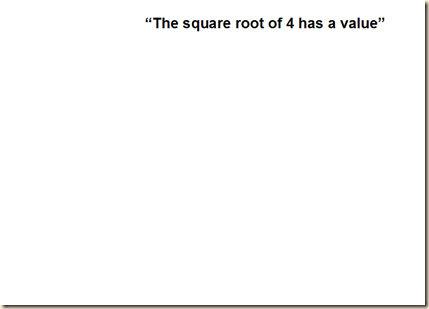It has a subject-predicate structure.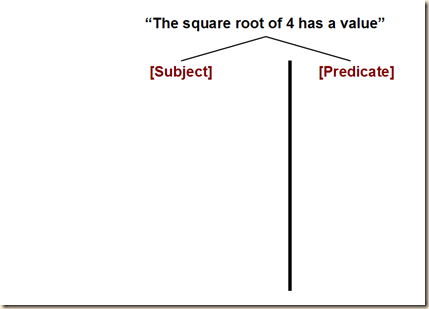The predicate is “has a value”.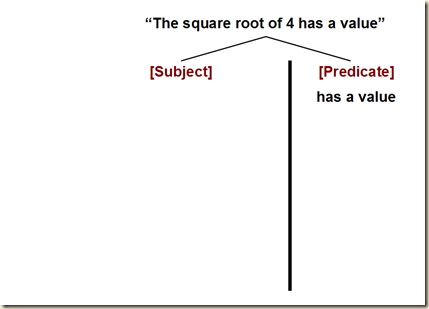The subject is “the square root of 4”.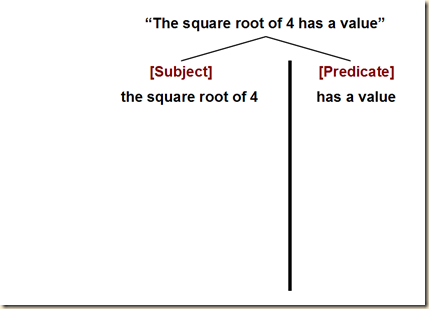The predicate “has a value” is conceptual.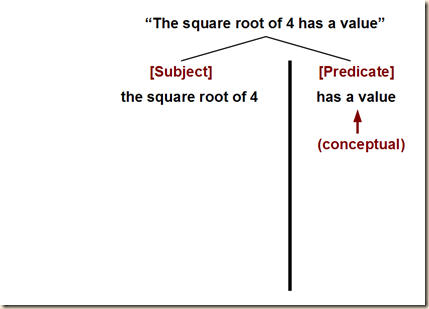And “the square root of 4” is a concept.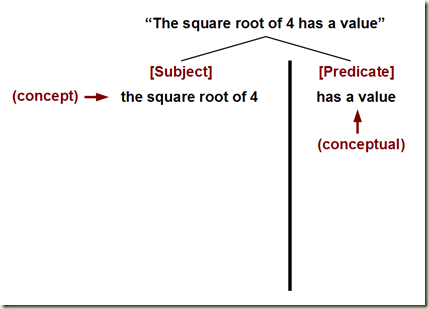But since “the square root of 4” is a concept, that means it also has a subject-predicate form.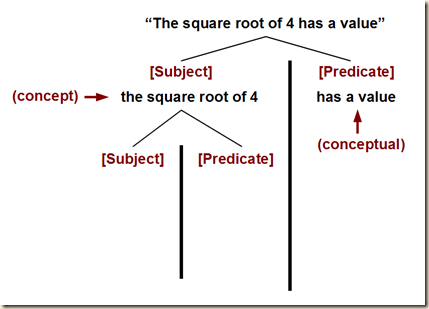The predicate is “is a square root of 4”.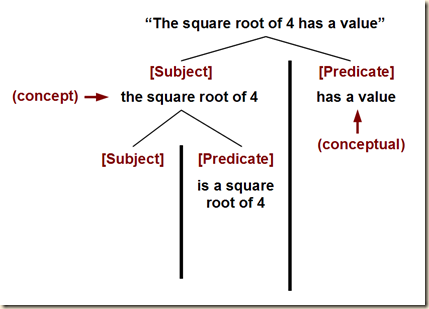And the subject perhaps is “the value in question (2 or –2, in fact)”.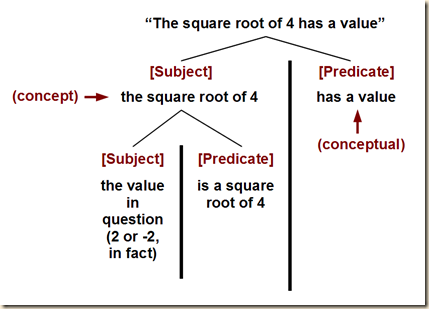The predicate here as always is conceptual.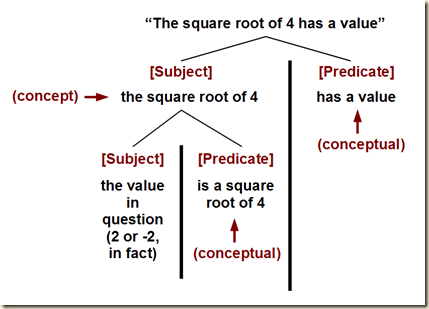But in this case, unlike the prior one, the subject is not a concept, but rather it is an object. So we do not continue dividing it into subject and predicate.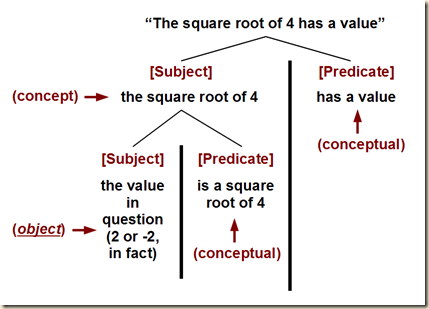]

But this does not in any way efface the distinction between object and concept. We see to | begin with that in the sentence ‘there is at least one square root of 4’ the predicative nature of the concept is not belied; we could say ‘there is something that has the property of giving the result 4 when multiplied by itself.’ Hence what is here asserted about a concept can never be asserted about an object; for a proper name can never be a predicative expression, though it can be part of one.

(49-50)

[So recall his example where we were not able to assert the same thing about the concept square root of 4 and the object “the concept of the square root of 4.”] Frege then clarifies that if we assert something about concept, and then make that same assertion about an object [that names this concept], we are not thereby saying something false. We are merely saying something senseless. He gives an example. [I think it works out like the following. Suppose we say, “there is a man whose name is Julius Caesar.” Let us try to break it down like the prior example. We have the subject-predicate structure of: /subject/ A man whose name is Julius Caesar /predicate/ exists (currently). (Here we might say that this can be true or false. It would be false if it were formulated to mean the Julius Caesar who is now dead, for example.) This is a concept-concept structure, because the subject is itself a concept. So this subject can be put in the subject- predicate structure: /subject/ the man in question (namely, the historical Julius Caesar) /predicate/ having the name ‘Julius Caesar’. This now is an object-concept structure. So again, we have the full sentence, “there is a man whose name is Julius Caesar,” and it both has sense and it can be true or false. However, Frege gives another example to compare with this one: “there is Julius Caesar.” Frege says that this sentence is neither true nor false, but rather is senseless. I am not sure exactly why, but let us look at what he is saying. He says that what we assert about a concept cannot be asserted about an object (presumably, that is, about a proper name standing for that same concept). So perhaps he is saying that in the first case we are clearly making a statement about the existence of some person. But in the second case, we are confusing things, because it would seem we are saying something about the existence of Julius Caesar as a concept. I am not sure. His other examples seem to re-illustrate the same point. He later gives an example with Cesar that has sense but is false “Julius Caesar is realized.” But the point there might be that the only kind of objects that can be realized are ones that take the form “the concept F’.  Let me quote:]

I do not want to say it is false to assert about an object what is asserted here about a concept; I want to say it is impossible, senseless, to do so. The sentence ‘there is Julius Caesar’ is neither true nor false but senseless; the sentence ‘there is a man whose name is Julius Caesar’ has a sense, but here again we have a concept, as the indefinite article shows. We get the same thing in the sentence ‘there is only one Vienna.’ We must not let ourselves be deceived because language often uses the same word now as a proper name, now as a concept-word; in our example, the numeral indicates that we have the latter; ‘Vienna’ is here a concept-word, like ‘metropolis.’ Using it in this sense, we may say: ‘Trieste is no Vienna.’ If, on the other hand, we substitute p. 2o1] ‘Julius Caesar’ for the proper name formed by the first six words of the sentence ‘the concept square root of 4 is realized,’ we get a sentence that has a sense but is false; for the assertion that something is realized (as the word is being taken here) can be truly made only about a quite special kind of objects, viz. such as can be designated by proper names of the form ‘the concept F.’ Thus the words ‘the concept square root of 4’ have an essentially different behaviour, as regards possible substitutions, from the words ‘square root of 4’ in our original sentence; i.e. the reference of the two phrases is essentially different.

(50)

Frege then says that although these are just a small number of examples, they illustrate a principle that holds more generally. Then he summarizes his main ideas from before, so let me quote them:

the behaviour of the concept is essentially predicative, even where something is being asserted about it; consequently it can be replaced there only by another concept, never by an object. Thus the assertion that is made about a concept does not suit an object. Second-level concepts, which concepts fall under, are essentially different from first-level concepts, which objects fall under. The relation of an object to a first-level concept that it falls under is different from the (admittedly similar) relation of a first-level to a second-level concept. (To do justice at once | to the distinction and to the similarity, we might perhaps say: An object falls under a first-level concept; a concept falls within a second-level concept.) The distinction of concept and object thus still holds, with all its sharpness.

(50-51)

Elsewhere Frege discussed his ideas of “property” and “mark”, and these concepts are coherently related to what he is saying now about concepts and objects. Regarding property, we can make a formulation like: “Φ is a property of Γ”. To use the notion of mark, we can say something like “Φ is a mark of Ω”. Frege claims that one same thing can be both a property and a mark, but it cannot be the property and the mark of one same thing. [Since Frege gives the example later, let us begin with it now, then after this discussion I will quote him in the order of his presentation. So consider these three statement: “2 is a positive number”, “2 is a whole number”, and “2 is less than 10”. Here we have three properties, and at the same time, three marks. The three properties are to be a positive number, to be a whole number, and to be less than 10. So recall Frege’s stipulation, this time I quote him: “a thing can be at once a property and a mark, but not of the same thing”. His point will be that these three properties are properties of the object 2, but they are not marks of 2. Rather, they are marks of something else, namely, of the concept positive whole number less than 10. This is evinced by the fact that this concept itself positive whole number less than 10 is not itself positive, whole, or less than 10 (because it as a concept of a number is not itself a number). So let us skip back then to his more formal presentation. We begin with a concept. Under this concept “falls” other concepts, namely, its properties. Suppose object Γ has three properties: Φ, Χ, and Ψ. It seems we next find a symbol to represent all three properties, and we here select Ω. So Ω is still a property, only it is actually three properties. Now, what about Φ, Χ, and Ψ? What are they properties of? Are they properties of Ω? No, they are not, because Ω itself does not have these properties (just like the concept 10 is not itself a whole number, etc.). Rather, they are “marks” of Ω; that is to say, they are the constituent parts falling within Ω but Ω does not fall under these properties/concepts. Frege explains that for one thing to be the property of another thing is for the second thing to fall under the first.  However, although they are marks of Ω, they are nonetheless still properties. But they are properties of something different, namely, of the object Γ we began this example with. So we say that Γ falls under Φ, Χ, and Ψ, but Φ, Χ, and Ψ fall within Ω. Thus Φ, Χ, and Ψ are both properties and marks, but just not of the same thing (they are properties of Γ but marks of   Ω). Frege then ties this to his notion about higher-level concepts. Φ, Χ, and Ψ are first level concepts. This part I do not understand, and I will explain why. My problem has to do mainly with the fact that he uses the term “subordinate to”, which would seem to be equivalent to “falls under”, but it cannot be, given his wording. Γ falls under its property Φ, which is a first-level concept. But Ω cannot fall under Φ. Nonetheless, Ω is subordinate to Φ. I am not sure what this subordination is. Perhaps it is merely dependence for its constitution. At any rate, Ω cannot fall under a first-level property like Φ. It could only do so to a second- level concept. With these current examples I am not sure how to illustrate that. Let us look back at the prior example, “the square root of 4 has a value”. Here we have the properties, has a value and is the square root of 4. The point here might be that the square root of 4 is both a mark and a property, but just not of the same thing. It is a property of the numbers 2 and –2. So 2 and –2 fall under the concept the square root of 4. But it is not a mark for 2 and –2. It is rather a mark for “the concept of the square root of 4”. As such, it can fall under another concept, higher-level one, namely, has a value. Probably I have this wrong, which is why I will quote for your own interpretation:]

With this there hangs together what I have said (Grundlagen, §53) about my usage of the words ‘property’ and ‘mark’; Kerry’s discussion gives me occasion to revert once more to this. The words serve to signify relations, in sentences like ‘Φ is a property of Γ’ and ‘Φ is a mark of Ω.' In my way of speaking, a thing can be at once a property and a mark, but not of the same thing. I call the concepts under which an object falls its properties; thus

‘to be Φ is a property of Γ'

is just another way of saying:

‘Γ falls under the concept of a Φ.’

If the object Γ has the properties Φ, Χ, and Ψ, I may combine them into Ω; so that it is the same thing if I say that Γ has the p. 202] property Ω, or, that Γ has the properties Φ, Χ, and Ψ. I then call Φ, Χ, and Ψ marks of the concept Ω, and, at the same time, properties of Γ. It is clear that the relations of Φ to Γ and to Ω are quite different, and that consequently different terms are required. Γ falls under the concept Φ; but Ω which is itself a concept, cannot fall under the first-level concept Φ; only to a second-level concept could it stand in a similar relation. Ω is, on the other hand, subordinate to Φ.

Let us consider an example. Instead of saying:

‘2 is a positive number’ and

‘2 is a whole number’ and

‘2 is less than 10’

we may also say ‘2 is a positive whole number less than 10.’ |

Here

to be a positive number,

to be a whole number,

to be less than 10,

appear as properties of the object 2, and also as marks of the concept

positive whole number less than 10.

This is neither positive, nor a whole number, nor less than 10. It is indeed subordinate to the concept whole number, but does not fall under it.

(51-52)

Frege then quotes Kerry, who seems to be using Frege’s notions of mark and property (52).

But according to Frege, Kerry misunderstands the distinction. In the quotation, Kerry refers to “the number 4” and “ ‘the’ number 4”. [Kerry is also quoted as writing “ ‘the’ number 4 is likewise the result of additively combining 3 and 1”] . But Frege says that whatever this distinction is, it is not his concept-object distinction. Kerry wants to say that the number 4 is a concept, and ‘the’ number 4 is a concept- object. But this is really Frege’s distinction between sense and reference (52).

Frege then wants to compare two formulations based on Kerry’s text [I will enumerate them, because Frege seems to be referring to these two sentences later by their enumeration]:

(1) “the number 4 is the result of additively combining 3 and 1”  and

(2) “ ‘the’ number 4 is the result of additively combining 3 and 1”.

Frege asks, is the “is” in these cases either the predicative copula or the logical equation? (52) Frege then says we need to first remove the “the” before “result” (53a). [This is perhaps in accordance with his rule that we use the indefinite article for predicates/concepts.] Frege then says that now we can say that both “the number 4” and “ ‘the’ number 4” are objects falling under the concept result of additively combining 3 and 1. He next asks, what is the difference between these two objects? [I am not following this part so well, but it seems Frege wants to change Kerry’s manner of articulating the concepts so they fit Frege’s distinctions, while doing justice to the insight Kerry is trying to communicate. So it is possible that Frege puts this question aside, and assumes there is no difference between the two formulations. Or, in what follows, Frege shows what that difference is. I cannot tell at this point which Frege is doing.] Frege then restates what Kerry is apparently trying to say: “The number 4 has those properties, and those alone, which are marks of the concept: result of additively combining 3 and 1” (53). Frege then says we might express “the first of our two sentences” [see above enumerated sentences]: “To be a number 4 is the same as being a result of additive combination of 3 and 1” (53). In that case, Kerry probably meant to say, “ ‘The number 4 has those properties, and those alone, which are marks of the concept a number 4’. p. 204] (We need not here decide whether this is true.) The inverted commas around the definite article in the words ‘ “the” number 4’ could in that case be omitted”  (53). [I am a little confused here, because the formulation with the inverted commas around the “the” is the second formulation, but Frege I thought was talking just about the first one here. So I am lost here and in fact I am as well throughout this whole discussion of Kerry’s number 4 example. Perhaps Frege’s point now is that we do not need two different sentences. Rather, we just need to make one sentence with both usages of “number 4,” but in one case we use the indefinite article and in the other we use the definite article without scare quotes. I am guessing wildly.]

Frege then addresses the proper and improper uses of the definite article in these cases. We consider the sentence, “The number 4 is none other than the result of additively combining 3 and 1” (53), and we are concerned with the “the” in front of “result”. Frege says this “the” is justified if there is such a result of this combination, and if there is no more than one such result. When these conditions are fulfilled, the phrase “the result of additively combining 3 and 1” is then understood as a proper name. [Frege then refers to “both of our sentences”. I think he again is talking about the two formulations, one with the scare quotes around “the”, enumerated by us above. His idea seems to be that we will consider if both cases use the equative ‘is’. This would mean that we could put the subjects of both sentences into an equality, because they are both equated to the same predicate. In that case, there would after all be no distinction between “the concept 4” and  “ ‘the’ concept 4”, as Kerry would have it. I am not certain at all, but let me offer a possible interpretation here. Recall that these are the two sentences in question:

(1) “the number 4 is the result of additively combining 3 and 1”  and

(2) “ ‘the’ number 4 is the result of additively combining 3 and 1”.

Two main things are possible: 1) the “is” is predicative, or 2) the “is” is equative. According to our criteria, since there is only one result, and thus since there is in fact a result, the “the” in “the result of additively combining 3 and 1” is properly used. Were an “a” the proper term, then this would be a predication and thus the predicative use of “is”. But since “the” is correct, this is a name and thus the “is” is instead an equative is. But that means the equative “is” is used for both sentences. That furthermore means that we can equate their subjects. In that case, there is no distinction between “the concept 4” and  “ ‘the’ concept 4”. Hence, Kerry is contradicting himself.] [Because my interpretation cannot be trusted, I recommend the reader see pages 52-53. Normally I would quote it all, but perhaps that would be too much text. And it seems only to illustrate a point Frege has made other ways. Also, his point was not so obvious to me here.]

Frege says that Kerry should be allowed to use the words “concept” and “object” whatever way he chooses. But Kerry should also let Frege have the same privilege, and furthermore, to acknowledge that Frege has made a more useful distinction:

I do not at all dispute Kerry’s right to use the words ‘concept’ and ‘object’ in his own way, if only he would respect my equal right, and admit that with my use of terms I have got hold of a distinction of the highest importance.

(54)

Frege then acknowledges that he may not always be expressing the distinction in a consistent way. So he asks for some charity from the reader [Note, Graham Priest quotes this passage in his book One, p.7.]

I admit that there is a quite peculiar obstacle in the way of an understanding with my reader. By a kind of necessity of language, my expressions, taken literally, sometimes miss my thought; I mention an object, when what I intend is a concept. I fully realize that in such cases I was relying upon a reader who would be ready to meet me halfway – who does not begrudge a pinch of salt.

(54)

Frege then says that perhaps the reader will find Frege’s notion of a concept to be too “unmanageable”. Rather, they might instead prefer Kerry’s idea that something can be either a concept or an object, depending on whether or not it is falling under another concept. Frege says, however, that instead of eliminating the difficulty, it has only been shifted. [For his explanation here, we should recall Frege’s distinction between saturated and unsaturated functions from “Function and Concept”. A function is unsaturated when its argument is not specified, as when for example an empty placeholder or a variable symbol is given in the argument place. And it is saturated when some specific argument is supplied. Frege’s problem with considering something as being ambiguously or dually both a concept and an object is that we cannot understand the sense of a sentence unless the subject and predicate are linked by means of this saturation structure. But when we conceive the subject as being ambiguously or dually both a concept and object, then we have lost this saturation structure and thus as well the grounds for there to be sense in the formulation. In other words, for there to be sense, there needs to be the conceptual relation of a something falling under a predicate. There is saturation when an object falls under another concept. But there is also saturation when a concept falls under another concept. But in that case, the concept itself is thought to have this saturation structure of it being composed of some object (or concept) falling under a concept. Nowhere here is something said in the same sentence to be in one respect a concept and in another respect an object. “All mammals” is a concept falling under “have red blood”. And “all mammals” contains the object “things” falling under the concept “being a mammal”. But we do not, like Kerry does, say that something is an object insofar as it falls under a concept, and thus we do not say that  “all mammals” is both an object, as it falls under “has red blood” and is also a concept, as it itself involves a predication, that is, has another thing falling under it.]

Somebody may think that this is an artificially created difficulty; that there is no need at all to take account of such an unmanageable thing as what I call a concept; that one might, like Kerry, regard an object’s falling under a concept as a relation, in which the same thing could occur now as object, now as concept. p. 205] The words ‘object’ and ‘concept’ would then serve only to indicate the different positions in the relation. This may be done; but anybody who thinks the difficulty is avoided this way is very much mistaken; it is only shifted. For not all the parts of a thought can be complete; at least one must be ‘unsaturated,’ or predicative; otherwise they would not hold together. For example, the sense of the phrase ‘the number 2’ does not hold together with that of the expression ‘the concept prime number’ without a link. We apply such a link in the sentence ‘the number 2 falls under the concept prime number’; it is contained in the words ‘falls under,’ which need to be completed in two ways-by a subject and an accusative; and only because their sense is thus ‘unsaturated’ are they capable of serving as a link. Only when they have been supplemented in this twofold respect do we get a complete sense, a thought. I say that such words or phrases stand for a | relation. We now get the same difficulty for the relation that we were trying to avoid for the concept. For the words ‘the relation of an object to the concept it falls under’ designate not a relation but an object; and the three proper names ‘the number 2,’ ‘the concept prime number,’ ‘the relation of an object to a concept it falls under,’ hold aloof from one another just as much as the first two do by themselves; however we put them together, we get no sentence. It is thus easy for us to see that the difficulty arising from the ‘unsaturatedness’ of one part of the thought can indeed be shifted, but not avoided. ‘Complete’ and ‘unsaturated’ are of course only figures of speech; but all that I wish or am able to do here is to give hints.

(54-55)

Frege concludes by suggesting we look to his work Funktion und Begriff. For, here he discusses the concept of function, which carries with it the same problem.

It may make it easier to come to an understanding if the reader compares my work Function und Begriff [sic]. For over the question what it is that is called a function in Analysis, we come up against the same obstacle; and on thorough investigation it will be found that the obstacle is essential, and founded on the nature of our language; that we cannot avoid a certain inappropriateness of linguistic expression; and that there is nothing for it but to realize this and always take it into account.

(55)

Frege, Gottlob. “On Concept and Object”. Transl. P.T. Geach. In Translations from the Philosophical Writings of Gottlob Frege. Eds. P.T. Geach and Max Black. Oxford: Basil Blackwell, 1960, second edition (1952 first edition).

.